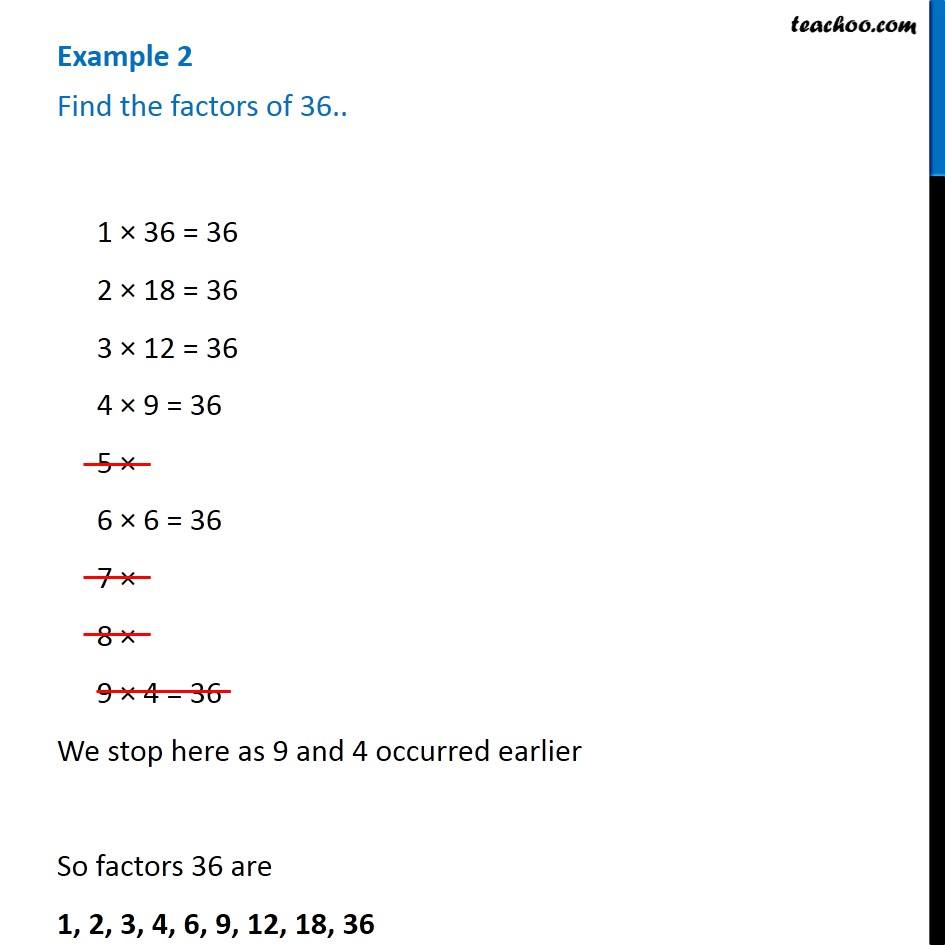Examples

Chapter 3 Class 6 Playing with Numbers
Serial order wiseThis video is only available for Teachoo black users

### Transcript

Example 2 Find the factors of 36..1 × 36 = 36 2 × 18 = 36 3 × 12 = 36 4 × 9 = 36 5 × 6 × 6 = 36 7 × 8 × 9 × 4 = 36 We stop here as 9 and 4 occurred earlier So factors 36 are 1, 2, 3, 4, 6, 9, 12, 18, 36